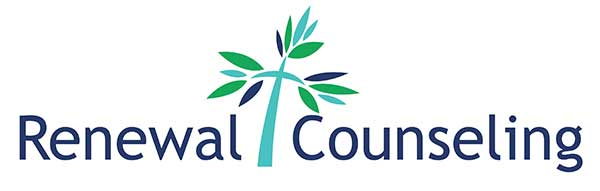jQuery(function(\$){var eqh_s=['.cst-intro-section .service-row .service_blurb','.service_blurb et_pb_blurb_description p'];var xb_eqh=function(h){try{var l=h.split(' ');if(l.length>0){var p='',c='',f=false;for(var i=0;i1){f=true;}}else{c+=l[i]+' ';}} if(c.length>0){\$(p).each(function(ip,ipl){\$(ipl).find(c).css('height','auto');var x=Math.max.apply(null,\$(ipl).find(c).map(function(){return \$(this).innerHeight();}).get());\$(ipl).find(c).each(function(h,t){\$(t).css("height",x)});});return;}} var a=\$(h);a.css('height','auto');var x=Math.max.apply(null,a.map(function(){return \$(this).innerHeight();}).get());a.each(function(h,t){\$(t).css("height",x)});}catch(e){}};try{eqh_s.forEach(function(i){xb_eqh(i);});\$(window).on('resize',function(){eqh_s.forEach(function(i){xb_eqh(i);});});}catch(e){}});
> Hours and Location

Hours and Location

Renewal Counseling125 Commerce Park Suite 102
Mooresville, NC 28117
Telephone: (704) 657-0195

Near intersection of Brawley School and HWY 21

Hours of Operation

• Mon
• Tue
• Wed
• Thur

*Fri – call for availability

Click here to get directions to our offices.